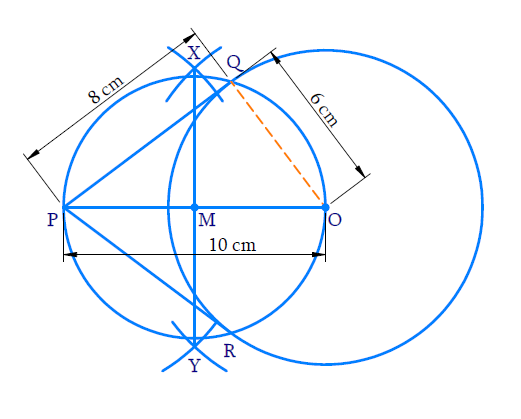# Ex.11.2 Q1 Constructions Solution - NCERT Maths Class 10

Go back to  'Ex.11.2'

## Question

Draw a circle of radius $$6 \,\rm{cm}$$. From a point $$10\,\rm{cm}$$ away from its centre, construct the pair of tangents to the circle and measure their lengths.

Video Solution
Constructions
Ex 11.2 | Question 1

## Text Solution

#### Steps:Steps of construction:

(i) Take a point $$O$$ as centre and $$6 \,\rm{cm}$$ radius. Draw a circle.

(ii) Take a point $$P$$ such that $$OP =$$ $$10\,\rm{cm}$$.

(iii) With $$O$$ and $$P$$ as centres and radius more than half of $$OP$$ draw arcs above and below $$OP$$ to intersect at $$X$$ and $$Y.$$

(iv) Join $$XY$$ to intersect $$OP$$ at $$M$$.

(v) With $$M$$ as centre and $$OM$$ as radius draw a circle to intersect the given circle at $$Q$$ and $$R$$.

(vi) Join $$PQ$$ and $$PR$$.

$$PQ$$ and $$PR$$ are the required tangents where\begin{align}\rm{PQ}=\rm{PR}=8 \rm{cm.}\end{align}

Proof:

$$\angle {{PQO}} = {90^ \circ } \Rightarrow {\rm{PQ}} \bot {\rm{OQ}}$$ (Angle in a semicircle)

$$OQ$$ being the radius of the given circle, $$PQ$$ in the tangent at $$Q.$$

In right $${{\Delta PQO,}}$$

$${{OP = 10 \,\rm{cm,} OQ = 6}}\,{\rm{cm}}$$ (radius)

\begin{align} {{P}}{{{Q}}^{{2}}} &={{O}}{{{P}}^{{2}}}{{ - O}}{{{Q}}^{{2}}}\\ &= {{ (10}}{{{)}}^{{2}}}{{ - (6}}{{{)}}^{{2}}}\\ &= { {100 - 36}}\\ &= {{ 64}} \end{align}

\begin{align} {{PQ }}&=\sqrt {{{64}}} \\ &= {{8}}\,{\rm{cm}} \end{align}

Similarly, $$PR =$$ $$8 \;\rm{cm}$$.

Learn from the best math teachers and top your exams

• Live one on one classroom and doubt clearing
• Practice worksheets in and after class for conceptual clarity
• Personalized curriculum to keep up with school